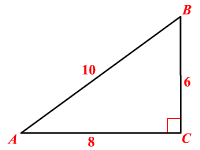# Tangent (of an angle)

The tangent of an angle is the trigonometric ratio between the adjacent side and the opposite side of a right triangle containing that angle.

$\text{tangent}=\frac{\text{length}\text{\hspace{0.17em}}\text{\hspace{0.17em}}\text{of}\text{\hspace{0.17em}}\text{\hspace{0.17em}}\text{the}\text{\hspace{0.17em}}\text{\hspace{0.17em}}\text{leg}\text{\hspace{0.17em}}\text{\hspace{0.17em}}\text{opposite}\text{\hspace{0.17em}}\text{\hspace{0.17em}}\text{to}\text{\hspace{0.17em}}\text{\hspace{0.17em}}\text{the}\text{\hspace{0.17em}}\text{\hspace{0.17em}}\text{angle}}{\text{length}\text{\hspace{0.17em}}\text{\hspace{0.17em}}\text{of}\text{\hspace{0.17em}}\text{\hspace{0.17em}}\text{the}\text{\hspace{0.17em}}\text{\hspace{0.17em}}\text{leg}\text{\hspace{0.17em}}\text{\hspace{0.17em}}\text{adjacent}\text{\hspace{0.17em}}\text{\hspace{0.17em}}\text{to}\text{\hspace{0.17em}}\text{\hspace{0.17em}}\text{the}\text{\hspace{0.17em}}\text{\hspace{0.17em}}\text{angle}}$ abbreviated as "tan"

Example:

In the triangle shown,

$\mathrm{tan}\left(A\right)=\frac{6}{8}\text{\hspace{0.17em}}\text{\hspace{0.17em}}\text{or}\text{\hspace{0.17em}}\text{\hspace{0.17em}}\frac{3}{4}$ and $\mathrm{tan}\left(B\right)=\frac{8}{6}\text{\hspace{0.17em}}\text{\hspace{0.17em}}\text{or}\text{\hspace{0.17em}}\text{\hspace{0.17em}}\frac{4}{3}$ .The tangent ratio is the same regardless of the size of the right triangle. So, it is often easiest to consider a right triangle with a hypotenuse of length $1$ .

The tangent ratio can also be thought of as a function, which takes different values depending on the measure of the angle. You can measure an angle in degrees or  radians .

The reciprocal of the tangent ratio is known as cotangent ratio of an angle, abbreviated as "cot".

That is,

$\text{cotangent}=\frac{\text{length}\text{\hspace{0.17em}}\text{\hspace{0.17em}}\text{of}\text{\hspace{0.17em}}\text{\hspace{0.17em}}\text{the}\text{\hspace{0.17em}}\text{\hspace{0.17em}}\text{leg}\text{\hspace{0.17em}}\text{\hspace{0.17em}}\text{adjacent}\text{\hspace{0.17em}}\text{\hspace{0.17em}}\text{to}\text{\hspace{0.17em}}\text{\hspace{0.17em}}\text{the}\text{\hspace{0.17em}}\text{\hspace{0.17em}}\text{angle}}{\text{length}\text{\hspace{0.17em}}\text{\hspace{0.17em}}\text{of}\text{\hspace{0.17em}}\text{\hspace{0.17em}}\text{the}\text{\hspace{0.17em}}\text{\hspace{0.17em}}\text{leg}\text{\hspace{0.17em}}\text{\hspace{0.17em}}\text{opposite}\text{\hspace{0.17em}}\text{\hspace{0.17em}}\text{to}\text{\hspace{0.17em}}\text{\hspace{0.17em}}\text{the}\text{\hspace{0.17em}}\text{\hspace{0.17em}}\text{angle}}$

In the above example, $\mathrm{cot}\left(A\right)=\frac{7}{4}$ and $\mathrm{cot}\left(B\right)=\frac{4}{7}$ .

See also: sine and cosine .